# How to Subtract Mixed Fractions with Unlike Denominators

Instructor: Mia Primas

Mia has taught math and science and has a Master's Degree in Secondary Teaching.

In this lesson, you will learn how to subtract mixed fractions with unlike denominators. The mixed fractions will be converted to improper fractions, then changed so that they have the same denominator.

## Steps for Subtracting Mixed Fractions with Unlike Denominators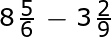• Step 1: Convert the mixed fractions to improper fractions.

Multiply the whole number by the denominator of the fraction and then add the numerator. This number becomes the numerator of the improper fraction. The denominator of the improper fraction remains the same as the denominator of the mixed fraction.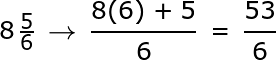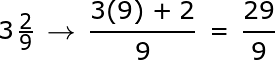• Step 2: Change the fractions so that they have the same denominator

We can make a common denominator by determining a common multiple of the denominators, 6 and 9. The multiples of 6 are 6, 12, 18, etc. The multiples of 9 are 9, 18, 27, etc. They have a common multiple of 18 which will be the new denominator for the fractions.

Multiplying the first fraction by 3/3 and the second fraction by 2/2 will result in 18 for both denominators. Note that 3/3 and 2/2 are equal to 1, so we are actually multiplying both fractions by 1 and not changing the value of the fractions in any way.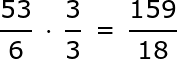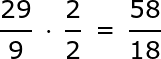• Step 3: Subtract the fractions

To unlock this lesson you must be a Study.com Member.

### Register to view this lesson

Are you a student or a teacher?

#### See for yourself why 30 million people use Study.com

##### Become a Study.com member and start learning now.
Back
What teachers are saying about Study.com

### Earning College Credit

Did you know… We have over 200 college courses that prepare you to earn credit by exam that is accepted by over 1,500 colleges and universities. You can test out of the first two years of college and save thousands off your degree. Anyone can earn credit-by-exam regardless of age or education level.